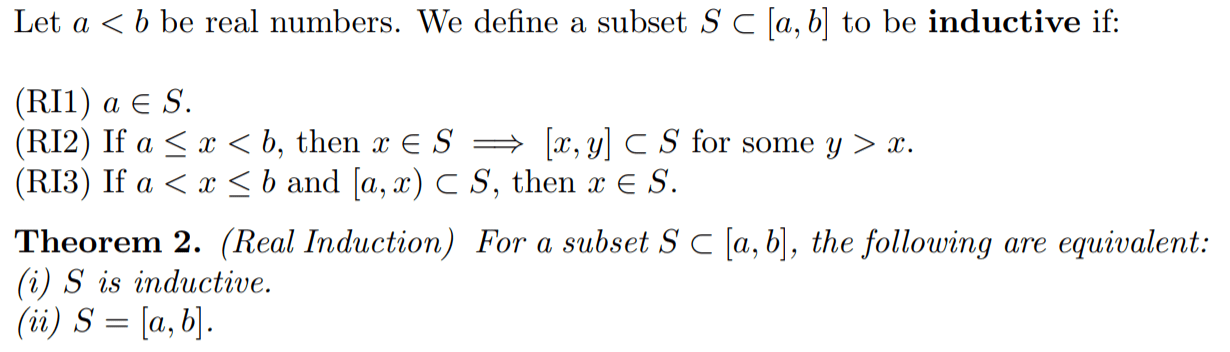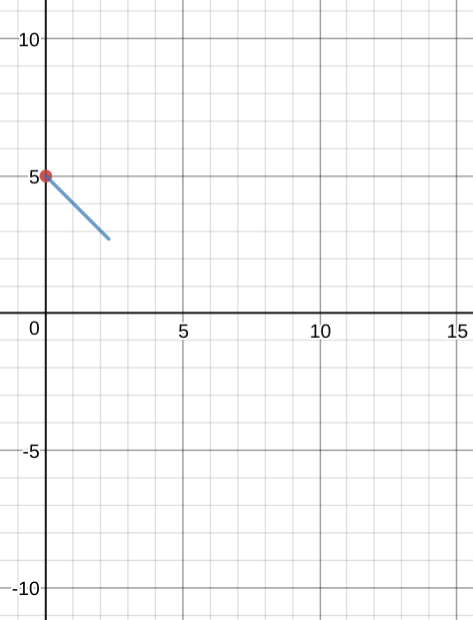# Continuous induction is a thing!

Great question:

From the paper:

Consider “conventional” mathematical induction. To use it, one thinks in terms of predicates — i.e., statements$P(n)$ indexed by the natural numbers — but the cleanest statement is in terms of subsets of$\mathbb{N}.$ The same goes for real induction.

I think of induction as being about dominoes falling, but the focus on sets is a different way of talking about induction — even for the natural numbers.

To be OK with this paper’s explanation of continuous induction, we have to be OK with the idea that its sets that the inductive property — it’s not a process metaphor, it’s a property metaphor, or something like that. Maybe a useful metaphor is flammability, rather than dominoes. Some sets are made of stone. Others you set a spark and the whole thing goes up in flames. Those are the inductive sets.

Here’s what it means for a set of real numbers to be inductive:The three conditions say what it means to be inductive, and the theorem states that if a set is inductive then it is fire: something that’s true for part of that inductive set has to be true for the whole thing.

(Something I’m wondering about real induction: can’t the spark can be planted either at the front or back of the set? I think with slightly different conditions for inductivity you could start this at b.)

The paper goes on to use this continuous induction to prove some of the classic theorems of calculus and analysis, including the Intermediate Value Theorem. Here’s my attempt to restate the proof in the paper.

So, I’m going to tell you three things about a graph. First, it starts like this and ends at 10:Second, the function is continuous.

Third, the function does not ever cross zero.

Question: Are you guaranteed that this graph stays positive the whole time?

Answer: Yes, duh. But as with a lot of this foundational stuff, the challenge is to say why.

So let’s make the following inductive argument, by first collecting all the inputs 0 through 10 of my function that yield positive outputs into a set. So far, just from the graph, you know that this set contains all the real numbers from 0 to about 2. We’d love to show that actually all the real numbers from 0 through 10 are part of this set.

Now, let’s tee up the conditions for this set being inductive.

1. 0 is in this set, because duh I showed you that 0 is in this set.
2. Pick any number that’s already in this set — it looks like 2.2 is in it — then there has to be some larger number in the set i.e. also yields a positive output. That’s because this function is continuous, and so approaching 2.2 from the right, eventually the outputs need to get really, really, REALLY close to the true output when 2.2 is the input to f. That necessarily means that it’s going to have a positive output, since the output of 2.2 is positive. So there needs to be numbers larger than 2.2 that are in this set if the function is continuous.
3. And suppose that you knew some range from 0 up to but not necessarily including 8 where was positive, i.e. still hanging out above that zero line. Well, even though we “but not necessarily including”-ed 8, 8 has got to be positive anyway. Because go backwards at all from 8 and you should hit positive outputs, but because this is continuous eventually all those positive values need to be (eventually) super-duper-duper close to$f(8)$. So 8 must have a positive output.

And by induction, tada, there’s nothing stopping you from extending this to the entire domain of 0 to 10, it’s positive the whole way through. And since this is just a restatement of the Intermediate Value Theorem, you’ve proven that too, by induction.

I still have questions, but this is very cool.